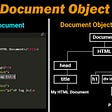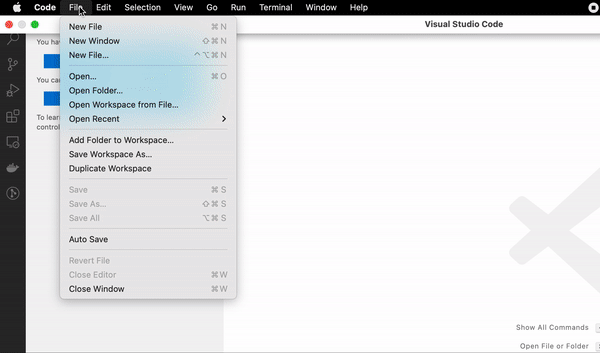# SVG

## How the Cubic Bezier curve is formed?

• Bezier control point #1 `(x1, y1)` and
• Bezier control point #2 `(x2, y2)`
`// Cubic Bezier path syntax<path D="M x0,y0  C x1,y1  x2,y2  x3,y3" />`
`size = 1000`

# Finding Coordinates

## Coordinate system & viewBox

`<svg viewBox="min-x min-y width height">...</svg>`

## Constant & Dynamic Coordinates

• `x0, y0`— First pair of anchor points that stays constant at all the times. Here, `x0` is the centre of the diagram `size` and `y0` is vertical point where the circle stops (…hence the addition of a radius) and path begins.
= `(50% of size, 20% of size + radius)`
• `x1, y1`— Bezier control point one, which also stays constant for all paths. Keeping symmetry in mind, `x1` and `y1` are always half of the diagram `size`.
= `(50% of size, 50% of size)`
• `x2, y2`— Bezier control point two, where `x2` directs which side to form the curve and is calculated dynamically for each paths. And again, `y2` will be half the diagram `size`.
= `(x2, 50% of size)`
• `x3, y3`- Final pair of anchor points that indicates where to stop drawing the path. Here, `x3` imitates the value of `x2`, which is to be calculated dynamically. And `y3` takes 80% of the `size`.
= `(x3, 80% of size)`
`<path d="M size*0.5, (size*0.2) + radius           C size*0.5,  size*0.5           x2,        size*0.5           x3,        size*0.8">`

## Finding Dynamic Coordinates (x2 and `x3`)

`distance = size/arrayLength// distance = 1000/5 = 200`
`// value of x2 and x3x = index * distance`
`x = index * distance + (distance * 0.5)`

`<defs>  <mask id="svg-mask">     <circle :r="radius"              :cx="halfSize"              :cy="topHeight"              fill="white"/>  </mask></defs>`
`<image mask="url(#svg-mask)"       :x="(halfSize-radius)"       :y="(topHeight-radius)"...> </image>`

# Dynamic SVG using Vue.js

• Binding SVG viewBox
• Calculating SVG Path Coordinates
• Two options for Bezier curve path implementation
• Config Panel
• Homework ❤
`viewbox() {   return "0 0 " + this.size + " " + this.size;}`
`<svg viewBox="0 0 1000 1000"></svg>`
`<svg :view-box.camel="viewbox">   ...</svg>`
• topHeight— `size * 0.2`
• bottomHeight — `size * 0.8`
• width — `size`
• halfSize — `size * 0.5`
• distance— `size/arrayLength`
`x = index * distance + (distance * 0.5)`

## Option 1

`<g v-for="(item, i) in itemArray">  <path :d="'M' + halfSize + ','         + (topHeight+r) +' '+            'C' + halfSize + ','         + halfSize +' '+                      calculateXPos(i) + ',' + halfSize +' '+                   calculateXPos(i) + ',' + bottomHeight"   /></g>`
`<script>  methods: {    calculateXPos (i)    {      return distance * i + (distance * 0.5)    }  }</script>`

## Option 2

`<g v-for="(item, i) in items">     <cubic-bezier  :index="i"                    :half-size="halfSize"                    :top-height="topHeight"                    :bottom-height="bottomHeight"                    :r="radius"                   :d="distance"     >     </cubic-bezier></g>`
`<clip-mask :title="title"           :half-size="halfSize"            :top-height="topHeight"                                :r="radius"> </clip-mask>`

# Homework

• Create a switch to toggle between horizontal and vertical mode
• Maybe use GSAP to animate the path
• Control path attributes (such as colour & stroke width) from config panel
• Use external library to save and download the diagram as an image/PDF

# Conclusion

--

--

--

## More from Krutie Patel

#Nuxtjs 👑 #Vuejs✌️#Laravel⚡️2D Illustrator✏️ 🎧 https://mymemoir.io/ 📙 https://www.pariksha.io/ 👑 https://krutiepatel.com/🐦 https://twitter.com/KrutiePatel

Love podcasts or audiobooks? Learn on the go with our new app.

## React: a JavaScript Library## React clean code, coding style guide, and best practice## Build an Instagram Clone With React.Js, Next.Js, and Bootstrap5 in 35 mins## Document Object Model## How to Apply a Filter to Angular for loop (*ngFor) in HTML## How to easily set up a static server with VS Code (NO backend knowledge required)## Setting up a component library with Github packages## Krutie Patel

#Nuxtjs 👑 #Vuejs✌️#Laravel⚡️2D Illustrator✏️ 🎧 https://mymemoir.io/ 📙 https://www.pariksha.io/ 👑 https://krutiepatel.com/🐦 https://twitter.com/KrutiePatel

## ReSvelte- A Svelte Developer Tool and Component Tree Visualizer (VS Code Extension)## Configuring Sonarqube in a Vite project, with Vue, Typescript and Vitest## Creating and Using TomTom Geofences with Vue## Ever wondered how to manage state in Svelte applications?